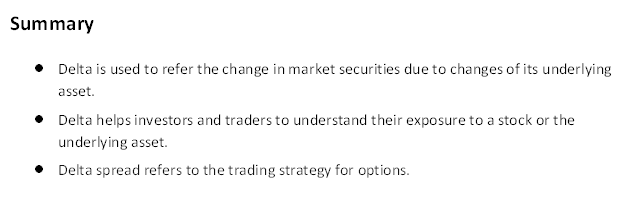# Delta

• Updated on

What is Delta?

Delta is a ratio that shows the change in price of derivatives price assets with the change in its underlying assets price. Delta is used at the time of dealing in options and as a hedge ratio.Understanding Delta

Delta is used to refer the change in market securities due to changes of its underlying asset. Delta represent as the value of an option’s price which move with the same amount of change in its underlying asset.

Traders use delta to understand the return and risk from an investment, for example A derivatives trader thinks a stock going to increase in near future by £1.5 per share, so he/she will buy a call option on the stock. Traders/ Investor use calculation of delta to calculate the return (potential gain) from the change in stocks. If a delta value is range from 0 to -1 it is called as put option and if value of delta is range from 0-1 it is called as call option. Delta can be calculated as:  Delta= ∂V/ ∂S

Where, ∂ stands for the first derivative, V stands for the option’s price, S stands for underlying asset’s price.

How does delta interpret?

Traders and investors use delta as a risk sensitivity measure of underlying asset price. It is a ratio that helps to understand the changes by comparing the changes in the underlying assets due to change in derivative price. The value of delta can be negative or positive; it depends on the direction in which derivative move due to change in underlying assets delta. Investors and traders understand its call option or put option with the ratio of delta. If underlying assets price would rise the value of call option will increase so we can be said that it has positive delta. If the put option has fall down with rise in the price of underlying assets than it said to be has negative delta.

In call option, delta value is move in the range of 0 to 1, and in put option, delta value move in the range of 0 to -1. Delta has positive or negative value is depend on the derivative whether it is a call or a put. For instance, assuming the value of a call option is £15, and £25 as the underlying asset. The underlying asset price rise to £28, in result option value rise to £16. So the delta can be calculated as (£16-£15) / (£28- £25), will get 0.33.

Now understand with a put option, it has a value of £15. And the price of underlying asset is £25. The underlying asset price fall to £22 and in result put option value rise to £16. The delta can be calculated here as (£16-£15) / (£22-£25) = -0.33.

Delta also helps investors and traders to understand whether an option will turn out to be end in the money at the time of expiry date. The delta value moves away from 0, if the option moves more into the money. In call option, it move to value of 1 and in put option it will move towards the value of -1.  Sensitivity of delta is affected by the time until its expiry.  By closing to the time of expiry, option can be end up whether in, out, or at the money.

Traders and investors use delta ratio at the time of determining the risk of any investment. By observing delta values, investors and traders may consider its values to understand their exposure to a stock or the underlying asset. It is helpful for the investors and traders to understand the changes in overall market by the portfolio aggregate delta. The delta value also helped investors or traders to take a decision about options whether they are being bought or sold. Trader will sell the option if the price of an option rises less than the delta implied, and trader will buy an option if the price is higher than the delta implied. Delta is also used for various purposes including:

• Delta can also be used as a hedging strategy.
• Delta is helpful for traders and investors to determine the risk they willing to take for an investment return.
• Delta is used to understand the changes in overall market by the portfolio aggregate delta.
• Delta helps traders to take decision of selling and buying an option.

What is the relation between delta and delta spread?

Delta spread refers to the trading strategy for options in which an investor or trader set up a delta neutral position in starting by buying and selling options in a proportion to the neutral ratio. In option trade, one of the most common tools which is used for implementation of delta spread strategy is calendar spread. Calendar spread is used for making a delta neutral position with the different expiry dates. Delta spread is used by traders and investors to make small profit in the situation of less fluctuation in the price of underlying security.

Talking about delta, it is used in delta spread for establishing a proportion to the neutral ratio. Neutral ratio is created by using positive and negative deltas offset each other. Delta spread is a trading started of options where, delta is used by investors to understand the changes in price of derivatives, price assets with the change in its underlying assets price.

##### Top ASX Listed Companies

Definition:
We use cookies to ensure that we give you the best experience on our website. If you continue to use this site we will assume that you are happy with it. OK